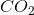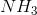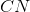# High School Chemistry : Help with VSEPR Theory and Geometry

## Example Questions

### Example Question #1 : Help With Vsepr Theory And Geometry

What is the molecular geometry of an ammonia molecule with the formula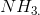?

Tetrahedral

Trigonal bipyramidal

Trigonal pyramidal

Trigonal planar

Trigonal pyramidal

Explanation:

In an ammonia molecule, the nitrogen is bonded to three hydrogen atoms and also has a lone electron pair. This lone pair will repel the three hydrogens out of a planar orientation, which results in a trigonal pyramidal geometry.

Compounds with the general formula AX3 and one lone pair will be trigonal pyramidal.

Compounds with the general formula AX3 and no lone pairs will be trigonal planar.

Compounds with the general formula AX4 and no lone pairs will be tetrahedral.

Compounds with the general formula AX5 and no lone pairs will be trigonal bipyramidal.

### Example Question #1 : Help With Vsepr Theory And Geometry

What is the bond angle found in a methane molecule with the formula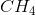?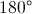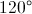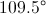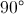Explanation:

Methane has a carbon atom attached to four hydrogen atoms. In order to be as far as possible from one another, the hydrogen atoms will orient themselves around the carbon in a tetrahedral geometry. Tetrahedral geometries have bond angles of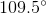between each constituent.

### Example Question #1 : Help With Vsepr Theory And Geometry

Which of the following choices correctly describes the VSEPR shape of the water molecule,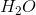?

Tetrahedral

Trigonal bipyramidal

Linear

None of the answer choices correctly describe the VSEPR shape of the water molecule

Trigonal planar

Tetrahedral

Explanation:

In the water molecule, there are four electron pairs. Two of them are bonded and two of them are lone pairs. This causes the water molecule to have a tetrahedral shape (it is important to note that it is a bent tetrahedral shape due to the two lone pairs).

### Example Question #101 : Elements And Compounds

Which of the following compounds has a bent shape according to VSEPR theory?